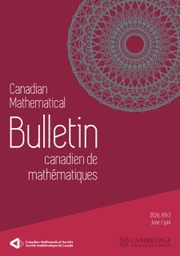Home
Hostname: page-component-768dbb666b-9qwsl Total loading time: 1.026 Render date: 2023-02-03T11:50:43.497Z Has data issue: true Feature Flags: { "useRatesEcommerce": false } hasContentIssue trueCanadian Mathematical Bulletin

## Abstract

We prove that the Riemann hypothesis is equivalent to the condition$\int _{2}^x\left (\pi (t)-\operatorname {\textrm {li}}(t)\right )\textrm {d}t<0$ for all$x>2$ . Here,$\pi (t)$ is the prime-counting function and$\operatorname {\textrm {li}}(t)$ is the logarithmic integral. This makes explicit a claim of Pintz. Moreover, we prove an analogous result for the Chebyshev function$\theta (t)$ and discuss the extent to which one can make related claims unconditionally.

Type
Article
Information

## Access options

Get access to the full version of this content by using one of the access options below. (Log in options will check for institutional or personal access. Content may require purchase if you do not have access.)

## References

Bays, C. and Hudson, R. H., A new bound for the smallest x with$\pi (x)>\textsf{li}(x)$ . Math. Comp. 69(2000), 12851296.CrossRefGoogle Scholar
Bennett, M., Martin, G., O’Bryant, K., and Rechnitzer, A., Explicit bounds for primes in arithmetic progressions . Illinois J. Math. 62(2018), nos. 1–4, 427532.CrossRefGoogle Scholar
Brent, R., Platt, D., and Trudgian, T., Accurate estimation of sums over zeros of the Riemann zeta-function . Math. Comp. 90(2021), 29232935.CrossRefGoogle Scholar
Broughan, K., Equivalents of the Riemann hypothesis. Volume 1: arithmetic equivalents, Cambridge University Press, Cambridge, 2017.Google Scholar
Büthe, J., On the first sign change in Mertens’ theorem . Acta Arith. 171(2015), no. 2, 183195.CrossRefGoogle Scholar
Büthe, J., An analytic method for bounding$\psi (x)$ . Math. Comp. 87(2018), no. 312, 19912009.CrossRefGoogle Scholar
Edwards, H. M., Riemann’s zeta function, Dover Publications, New York, 1974.Google Scholar
Ford, K., Zero-free regions for the Riemann zeta function . In: Number theory for the millennium, II (Urbana, IL, 2000), A K Peters, Natick, MA, 2002, pp. 2556.Google Scholar
Garcia, S. R. and Lee, E. S., Unconditional explicit Mertens’ theorems for number fields and Dedekind zeta residue bounds . Ramanujan J. 57(2022), no. 3, 11691191.CrossRefGoogle Scholar
Granville, A. and Martin, G., Prime number races . Amer. Math. Monthly 113(2006), no. 1, 133.CrossRefGoogle Scholar
Humphries, P., The distribution of weighted sums of the Liouville function and Pólya’s conjecture . J. Number Theory 133(2013), no. 2, 545582.CrossRefGoogle Scholar
Ingham, A. E., The distribution of prime numbers, Cambridge University Press, New York, 1964.Google Scholar
Kaczorowski, J., On sign-changes in the remainder-term of the prime number formula, I . Acta Arith. 44(1984), 365377.CrossRefGoogle Scholar
Karatsuba, A. A., On the Riemann asymptotic formula for$\pi (x)$ . Dokl. Math. 69(2004), no. 3, 423424.Google Scholar
Lamzouri, Y., A bias in Mertens’ product formula . Int. J. Number Theory 12(2016), no. 1, 97109.CrossRefGoogle Scholar
Littlewood, J. E., Sur la distribution des nombres premiers . CR Acad. Sci. Paris 158(1914), no. 1914, 18691872.Google Scholar
Martin, G., Mossinghoff, M. J., and Trudgian, T. S., Fake mu’s. Preprint, 2021. arXiv:2112.05227Google Scholar
Mossinghoff, M. J. and Trudgian, T. S., Nonnegative trigonometric polynomials and a zero-free region for the Riemann zeta-function . J. Number Theory 157(2015), 329349.CrossRefGoogle Scholar
Pintz, J., On an assertion of Riemann concerning the distribution of prime numbers . Acta Math. Hungar. 58(1991), nos. 3–4, 383387.CrossRefGoogle Scholar
Platt, D. J. and Trudgian, T. S., On the first sign change of$\theta (x)-x$ . Math. Comp. 85(2016), no. 299, 15391547.CrossRefGoogle Scholar
Riemann, B., Ueber die Anzahl der Primzahlen unter einer gegebenen Grosse . Ges. Math. Werke und Wissenschaftlicher Nachlaß. 2(1859), 145155.Google Scholar
Rosser, J. B. and Schoenfeld, L., Approximate formulas for some functions of prime numbers . Illinois J. Math. 6(1962), no. 1, 6494.CrossRefGoogle Scholar
Rubinstein, M. and Sarnak, P., Chebyshev’s bias . Exp. Math. 3(1994), no. 3, 173197.CrossRefGoogle Scholar
Saouter, Y., Trudgian, T., and Demichel, P., A still sharper region where$\pi (x)- li(x)$ is positive. Math. Comp. 84(2015), no. 295, 24332446.Google Scholar
Schmidt, E., Über die Anzahl der Primzahlen unter gegebener Grenze . Math. Ann. 57(1903), no. 2, 195204.CrossRefGoogle Scholar
Stechkin, S. B. and Popov, A. Y., The asymptotic distribution of prime numbers on the average . Russian Math. Surveys 51(1996), 6.CrossRefGoogle Scholar

# Save article to Kindle

Note you can select to save to either the @free.kindle.com or @kindle.com variations. ‘@free.kindle.com’ emails are free but can only be saved to your device when it is connected to wi-fi. ‘@kindle.com’ emails can be delivered even when you are not connected to wi-fi, but note that service fees apply.

Find out more about the Kindle Personal Document Service.

On the average value of$\pi (t)-\operatorname {\textrm {li}}(t)$Available formats × # Save article to Dropbox To save this article to your Dropbox account, please select one or more formats and confirm that you agree to abide by our usage policies. If this is the first time you used this feature, you will be asked to authorise Cambridge Core to connect with your Dropbox account. Find out more about saving content to Dropbox. On the average value of$\pi (t)-\operatorname {\textrm {li}}(t)$
Available formats
×

# Save article to Google Drive

To save this article to your Google Drive account, please select one or more formats and confirm that you agree to abide by our usage policies. If this is the first time you used this feature, you will be asked to authorise Cambridge Core to connect with your Google Drive account. Find out more about saving content to Google Drive.

On the average value of$\pi (t)-\operatorname {\textrm {li}}(t)\$
Available formats
×
×